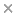BaseSpace
Correlation Engine 2.0Search sequence regions

###### filter terms:
• metric (15)
• Sizes of these terms reflect their relevance to your search.

In many classification scenarios, the data to be analyzed can be naturally represented as points living on the curved Riemannian manifold of symmetric positive-definite (SPD) matrices. Due to its non-Euclidean geometry, usual Euclidean learning algorithms may deliver poor performance on such data. We propose a principled reformulation of the successful Euclidean generalized learning vector quantization (GLVQ) methodology to deal with such data, accounting for the nonlinear Riemannian geometry of the manifold through log-Euclidean metric (LEM). We first generalize GLVQ to the manifold of SPD matrices by exploiting the LEM-induced geodesic distance (GLVQ-LEM). We then extend GLVQ-LEM with metric learning. In particular, we study both 1) a more straightforward implementation of the metric learning idea by adapting metric in the space of vectorized log-transformed SPD matrices and 2) the full formulation of metric learning without matrix vectorization, thus preserving the second-order tensor structure. To obtain the distance metric in the full LEM learning (LEML) approaches, two algorithms are proposed. One method is to restrict the distance metric to be full rank, treating the distance metric tensor as an SPD matrix, and readily use the LEM framework (GLVQ-LEML-LEM). The other method is to cast no such restriction, treating the distance metric tensor as a fixed rank positive semidefinite matrix living on a quotient manifold with total space equipped with flat geometry (GLVQ-LEML-FM). Experiments on multiple datasets of different natures demonstrate the good performance of the proposed methods.

### Citation

Fengzhen Tang, Peter Tino, Haibin Yu. Generalized Learning Vector Quantization With Log-Euclidean Metric Learning on Symmetric Positive-Definite Manifold. IEEE transactions on cybernetics. 2022 Jun 14;PP

PMID: 35700257

View Full Text